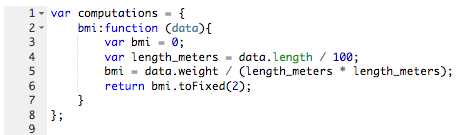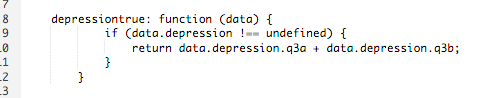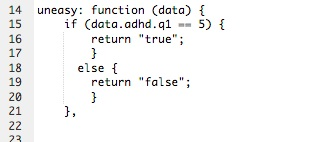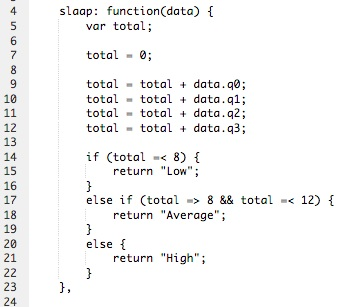What are good names for my questions and values?

The more complicated the computation, the more important you come up with good names for your questions. This way, others can also quickly understand what exactly is being calculated.

A good rule of thumb when naming questions is: choose something that is easily recognizable, makes sense and make it as short and simple as possible.

Examples of good names

Think about naming your questions before you start making your document. This will save time and frustration later on!

 Fieldname Use the name of the questionnaire or subject, e.g. 'BDI', 'adhd' or 'mood' when working with a choices. When you're working with a lot of separate (choice) questions that belong together thematically and you want to add up, simply use 'q1' or 'q2'.  When there are multiple questionnaires with separate questions in one document that have different themes, use for example 'BDI_q1', 'BDI_q2', and 'adhd_q1', etc. to differentiate. Question value For a choices, it is best to use use 'q1', 'q2' if you are going to do a sum. Option value For a set or a choices, use number values (integers): 1, 2, 3 or for a boolean: 'true' and 'false' or 'yes' and 'no'.

See the example of a multiple choice question below.How do I rescale a question?

There are 2 ways to rescale a question.

If you want to limit the number of questions in your computation, it is most convenient to subtract the answer from the maximum value on the question. See the example below.If you subtract the answer value from the maximum value (and the minimum value is 0), you rescale the question. Suppose your maximum value is 4:

 4 - 4 = 0 4 - 3 = 1 4 - 2 = 2 4 - 1 = 3 4 - 0 = 4

2 Create a "rescale" variable

You can also create a "rescale" variable. This is a bit more complicated and will be used if you want something other than simply flipping values.

In the example below you see a rescale variable where someone who fills in a 0 or 1 gets a score of 0. If someone enters a 2 or 3, that person gets a score of 1.So you build a variable "rescale" in which you describe how the values should be changed. You then refer to this in the questions that need to be scaled up using "this.rescale (data.q #)".

How do you calculate the BMI?

Length is expressed in centimeters, so first needs to be divided by 100 into length_meters. Then you start the BMI calculation using the variable length_meters we have just defined. See the example below.The code “.toFixed(2)” explained: If you want the answer to be fixed to 2 decimal points, add this to your return variable name.

How do you make if-then formulations?

An if-then statement adds many possibilities to personalize your computation. When would you use an if-else statement?

• When you want your computation to give back different results (for example 'high' and 'low' or 'true' and 'false') based on one, two, or more conditions.
• When you want conditional content to appear on basis of more than two conditions.
• When you want to make (advanced) rescalers, where solely reversal of values is not enough, for example when you want to give back 1 when someone scores 0-3 and 2 when someone scores 4-6.

There are three different if-else statements:

1. The if-statement

Here you define 1 condition and 1 result that is returned based on that condition. You can use the code as follows:

If (condition) {

action to perform ;

}

For example: 'if (data.questionnaire.q1 == 1) {return true; }'2. The if-else statement

Here you define 1 condition and 2 results, 1 based on the condition stated and 1 alternate result that is returned in ALL other cases. You can use the code as follows:

If (condition) {

action to perform ;

}

else {

alternate action to perform ;

}3. The if, if-else statement

Here you define 2 conditions and 3 results, 2 based on the conditions stated and 1 that is returned in ALL other cases. This is handy when you want to make a decision tree, where different answers to 1 question are returned to different categories. You can use the code as follows:

If (condition) {

action to perform ;

}

else if (alternate condition) {

alternate action to perform ;

}

else (condition in all other cases) {

action to perform ;

}As you can see in the picture, you can place multiple if, if-else statements one after the other.

How can you randomize?

If you want to divide your participants into an experimental and control group randomly (for example, before assigning different treatments), you can use a Math.random() computation for this. It goes like this:

experimental_or_control: function(data) {

data = data;

if (Math.random() <= 0.5) {

return "experimental";

} else {

return "control";

}

}

Once you have built this, you can trigger different content for either the experimental group or the control group with a trigger. Then use "computation: experimental_or_control" with your trigger.